# Excel for Finance

The top 10 Excel functions you need to know

## Guide to Excel for Finance – Functions & Formulas

If you want to learn Excel for finance, then you’ve come to the right spot.  At CFI, we’re on a mission to help you advance your career as a financial analyst…and being an expert at Excel is a big part of that.

In this guide, we’ve broken down what we believe are the most important Excel functions for finance professionals.  If you work your way through this list, we’re confident you’ll be well prepared to be a world-class financial analyst in Excel.

### Top 10 Excel Functions for Finance

Here are the top 10 most important functions and formulas you need to know, plain and simple.  Follow this guide and you’ll be ready to tackle any financial problems in Excel.  It should be noted that while each of these formulas and functions are useful independently, they can also be used in combinations that make them even more powerful.  We will point out these combinations wherever possible.

### #1 XNPV

Formula: =XNPV(discount_rate, cash_flows, dates)

The number one formula in Excel for finance professionals has to be XNPV.  Any valuation analysis aimed at determining what a company is worth will need to determine the Net Present Value (NPV) of a series of cash flows.

Unlike the regular NPV function in Excel, XNPV takes into account specific dates for cash flows and is, therefore, much more useful and precise.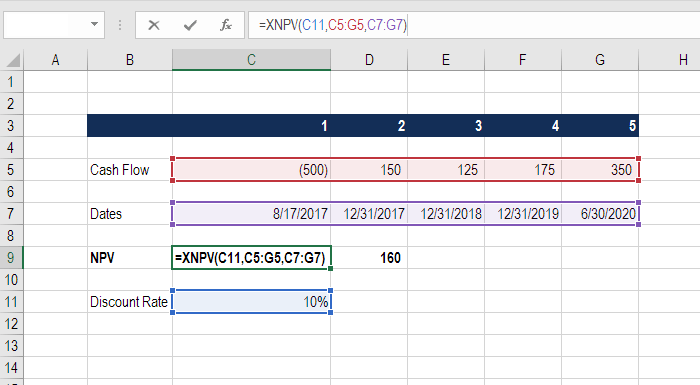### #2 XIRR

Formula: =XIRR(cash flows, dates)

Closely related to XNPV, another important function is XIRR, which determines the internal rate of return for a series of cash flows, given specific dates.

XIRR should always be used over the regular IRR formula, as the time periods between cash flows are very unlikely to all be exactly the same.

To learn more, see our guide comparing XIRR vs IRR in Excel.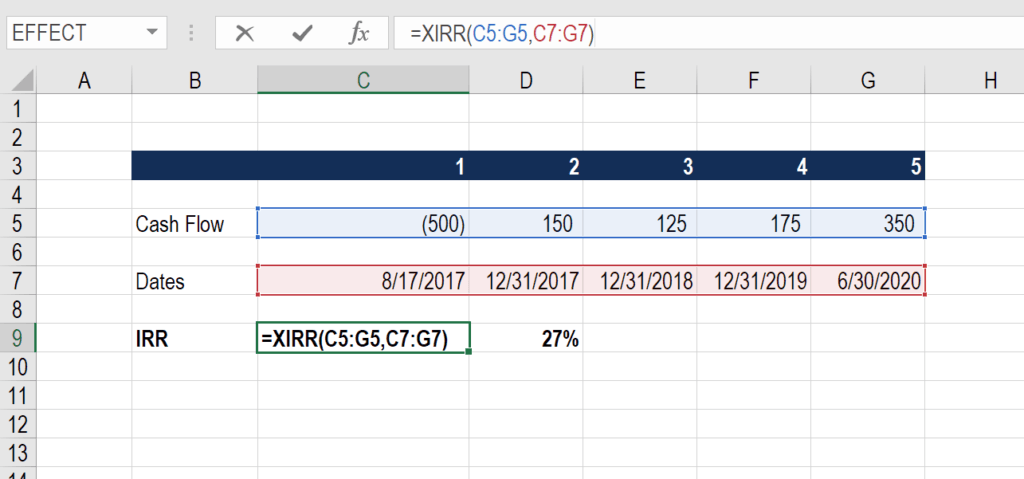### #3 MIRR

Formula: =MIRR(cash flows, cost of borrowing, reinvestment rate)

Here is another variation of the internal rate of return that’s very important for finance professionals.  The M stands for Modified, and this formula is particularly useful if the cash from one investment is invested in a different investment.

For example, imagine if the cash flow from a private business is then invested in government bonds.

If the business is high returning and produces an 18% IRR, but the cash along the way is reinvested in a bond at only 8%, the combined IRR will be much lower than 18% (it will be 15%, as shown in the example below).

Below is an Example of MIRR in action.### #4 PMT

Formula: =PMT(rate, number of periods, present value)

This is a very common function in Excel for finance professionals working with real estate financial modeling.  The formula is most easily thought of as a mortgage payment calculator.

Given an interest rate, and a number of time periods (years, months, etc.) and the total value of the loan (e.g., mortgage) you can easily figure out how much the payments will be.

Remember this produces the total payment, which includes both principal and interest.

See an example below that shows what the annual and monthly payments will be for a \$1 million mortgage with a 30-year term and a 4.5% interest rate.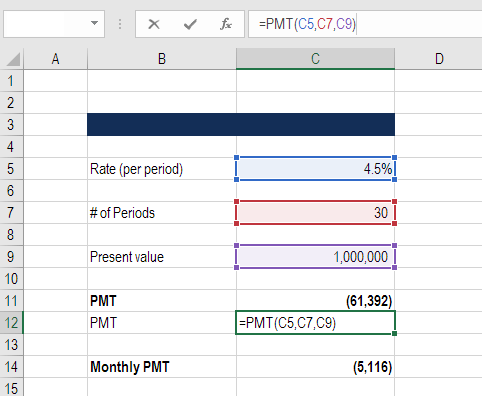### #5 IPMT

Formula: = IPMT(rate, current period #, total # of periods, present value)

IPMT calculates the interest portion of a fixed debt payment.  This Excel function works very well in conjunction with the PMT function above.  By separating out the interest payments in each period, we can then arrive at the principal payments in each period by taking the difference of PMT and IMPT.

In the example below, we can see that the interest payment in year 5 is \$41,844 on a 30-year loan with a 4.5% interest rate.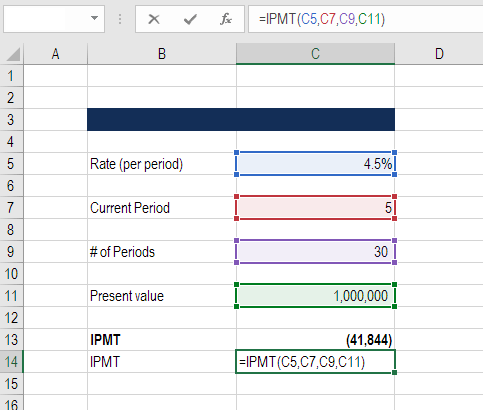### #6 EFFECT

Formula: =EFFECT(interest rate, # of periods per year)

This finance function in Excel returns the effective annual interest rate for non-annual compounding.  This is a very important function in Excel for finance professionals, particularly those involved with lending or borrowing.

For example, a 20.0% annual interest rate (APR) that compounds monthly is actually a 21.94% effective annual interest rate.

See a detailed example of this Excel function below.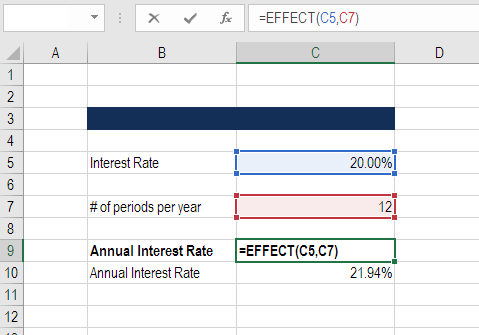### #7 DB

Formula: =DB(cost, salvage value, life/# of periods, current period)

This is a great Excel function for accountants and finance professionals.  If you want to avoid building a large Declining Balance (DB) depreciation schedule, Excel can calculate your depreciation expense in each period with this formula.

Below is an example of how to use this formula to determine DB depreciation.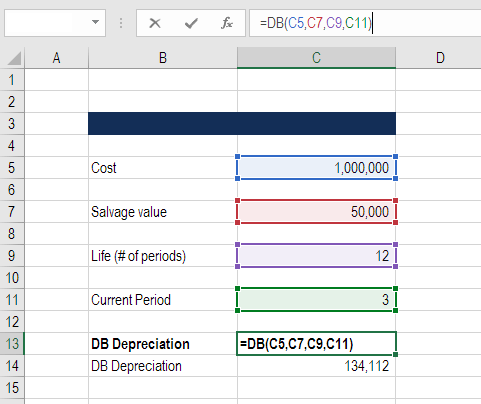In financial modeling, analysts typically build out a depreciation schedule manually.  To learn more, check out our series of financial modeling courses.

### #8 RATE

Formula: =RATE(# of periods, coupon payment per period, price of bond, face value of bond, type)

The RATE function can be used to calculate the Yield to Maturity for a security.  This is useful when determining the average annual rate of return that is earned from buying a bond.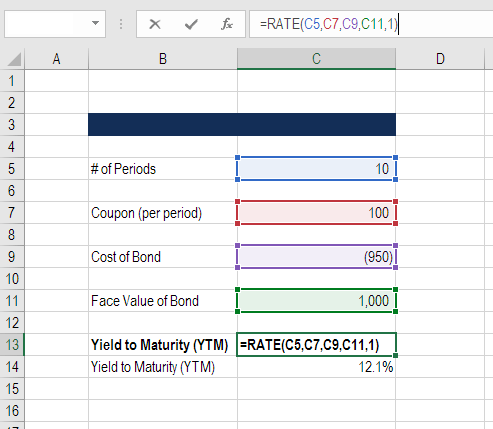### #9 FV

Formula: =FV(rate, # of periods, payments, starting value, type)

This function is great if you want to know how much money you will have in the future, given a starting balance, regular payments, and a compounding interest rate.

In the example below, you will see what happens to \$25 million if it’s grown at 4.5% annually for 30 years and receives \$1 million per year in additions to the total balance.  The result is \$154.6 million.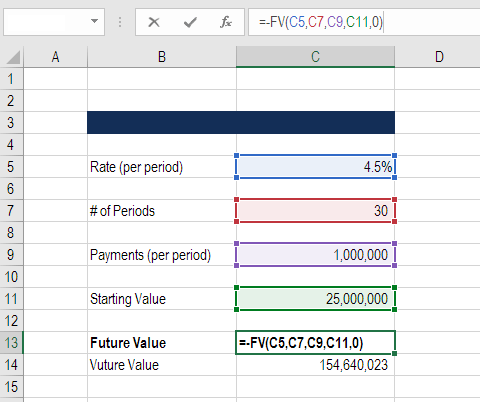### #10 SLOPE

Formula: =SLOPE(dependent variable, independent variable)

Finance professionals often have to calculate the Beta (volatility) of a stock when performing valuation analysis and financial modeling.  While you can grab a stock’s Beta from Bloomberg or from CapIQ, it’s often the best practice to build the analysis yourself in Excel.

The slope function in Excel allows you to easily calculate Beta, given the weekly returns for a stock and the index you wish to compare it to.

The example below shows exactly how to calculate beta in Excel for financial analysis.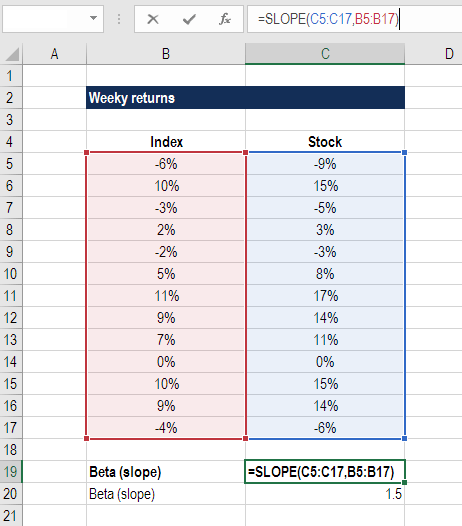### More Excel for Finance resources

We hope this has been a helpful guide to Excel for Finance. At CFI we are on a mission to help every financial analyst be world-class.  The CFI resources below will be extremely valuable in helping you along this path.

Relevant resources include: